# 一、树状结构

## 2、树结构概念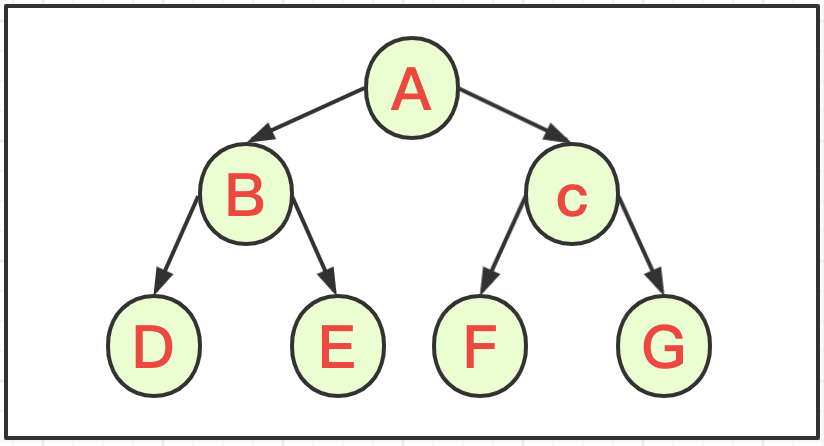• 根节点：树的根源，没有父节点的节点，如上图A节点；
• 兄弟节点：拥有同一父节点的子节点。如图B与C点；
• 叶子节点：没有子节点的节点。如图DEFG节点；
• 树的高度：最大层数，如图为3层；
• 路径：从root根节点找到指定节点的路线；

# 二、二叉树模型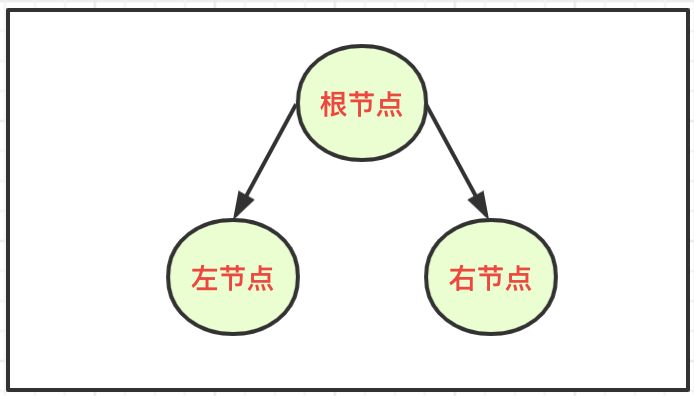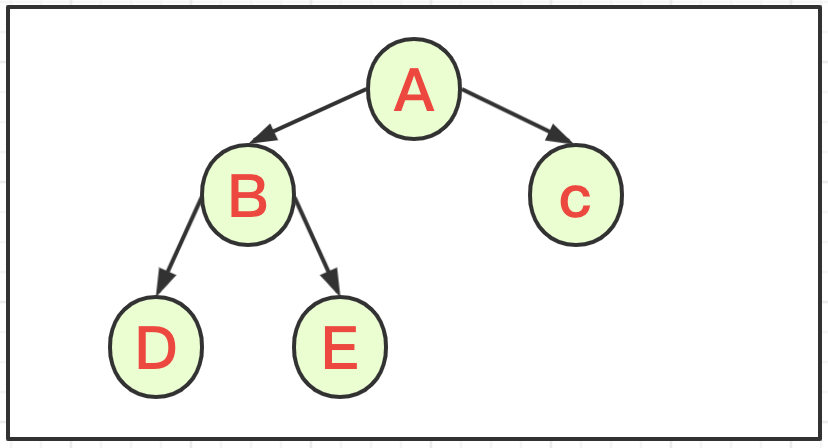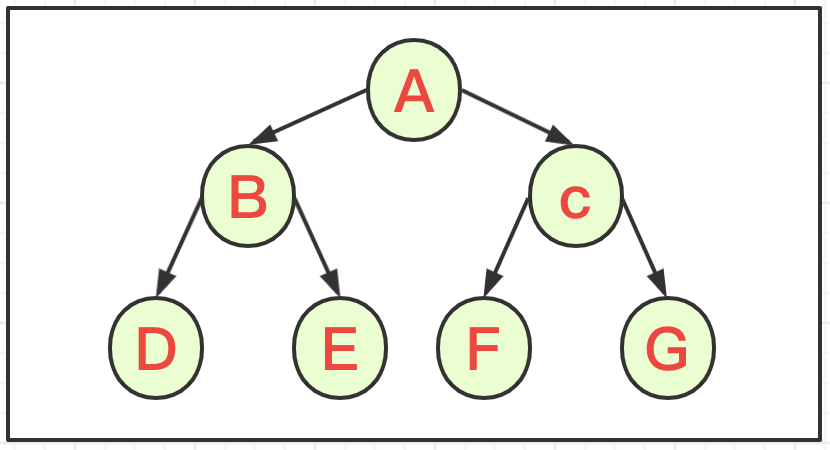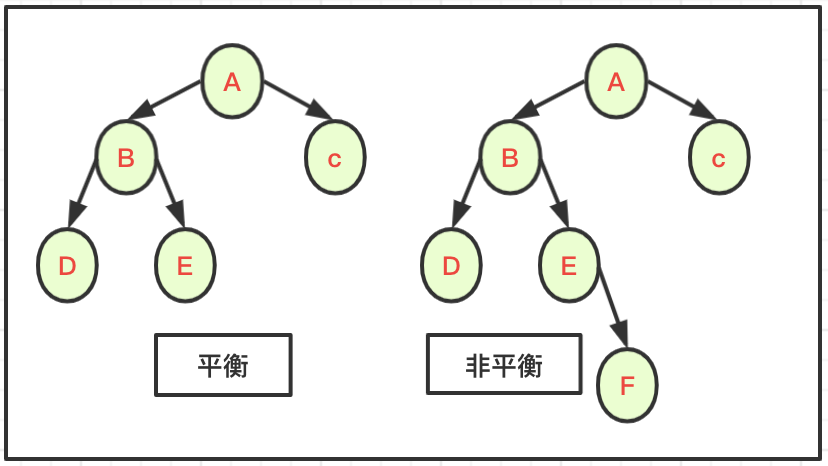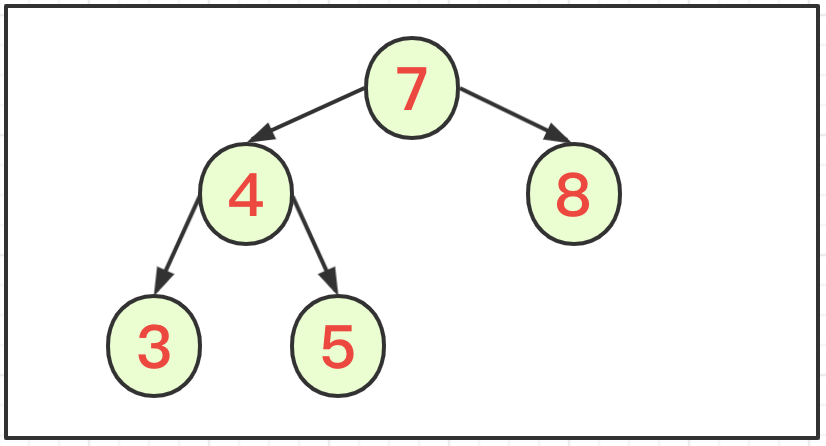# 三、二叉树编码

## 1、基础代码

class TreeNode {
private String num ;
private TreeNode leftNode ;
private TreeNode rightNode ;
public TreeNode(String num) {
this.num = num;
}
@Override
public String toString() {
return "TreeNode{num=" + num +'}';
}
}

class BinaryTree01 {
private TreeNode root ;
}

## 2、遍历与查找

public void prevTraverse() {
// 输出父结点
System.out.println(this);
// 向左子树递归前序遍历
if(this.leftNode != null) {
this.leftNode.prevTraverse();
}
// 向右子树递归前序遍历
if(this.rightNode != null) {
this.rightNode.prevTraverse();
}
}
public TreeNode prevSearch(String num) {
//比较当前结点
if(this.num.equals(num)) {
return this ;
}
// 递归遍历左子树查找
TreeNode findNode = null;
if(this.leftNode != null) {
findNode = this.leftNode.prevSearch(num);
}
// 左子树遍历命中
if(findNode != null) {
return findNode ;
}
// 递归遍历右子树查找
if(this.rightNode != null) {
findNode = this.rightNode.prevSearch(num);
}
return findNode ;
}

public void midTraverse() {
// 向左子树递归中序遍历
if(this.leftNode != null) {
this.leftNode.midTraverse();
}
// 输出父结点
System.out.println(this);
// 向右子树递归中序遍历
if(this.rightNode != null) {
this.rightNode.midTraverse();
}
}
public TreeNode midSearch(String num) {
// 递归遍历左子树查找
TreeNode findNode = null;
if(this.leftNode != null) {
findNode = this.leftNode.midSearch(num);
}
if(findNode != null) {
return findNode ;
}
// 比较当前结点
if(this.num.equals(num)) {
return this ;
}
// 递归遍历右子树查找
if(this.rightNode != null) {
findNode = this.rightNode.midSearch(num);
}
return findNode ;
}

public void lastTraverse() {
// 向左子树递归后序遍历
if(this.leftNode != null) {
this.leftNode.lastTraverse();
}
// 向右子树递归后序遍历
if(this.rightNode != null) {
this.rightNode.lastTraverse();
}
// 输出父结点
System.out.println(this);
}
public TreeNode lastSearch(String num) {
// 递归遍历左子树查找
TreeNode findNode = null;
if(this.leftNode != null) {
findNode = this.leftNode.lastSearch(num);
}
if(findNode != null) {
return findNode ;
}
// 递归遍历右子树查找
if(this.rightNode != null) {
findNode = this.rightNode.lastSearch(num);
}
if(findNode != null) {
return findNode ;
}
// 比较当前结点
if(this.num.equals(num)) {
return this ;
}
return null ;
}

## 3、删除节点

public void deleteNode(String num) {
// 判断左节点是否删除
if(this.leftNode != null && this.leftNode.num.equals(num)) {
this.leftNode = null ;
return ;
}
// 判断右节点是否删除
if(this.rightNode != null && this.rightNode.num.equals(num)) {
this.rightNode = null;
return ;
}
// 向左子树遍历进行递归删除
if(this.leftNode != null) {
this.leftNode.deleteNode(num);
}
// 向右子树遍历进行递归删除
if(this.rightNode != null) {
this.rightNode.deleteNode(num);
}
}

# 四、多叉树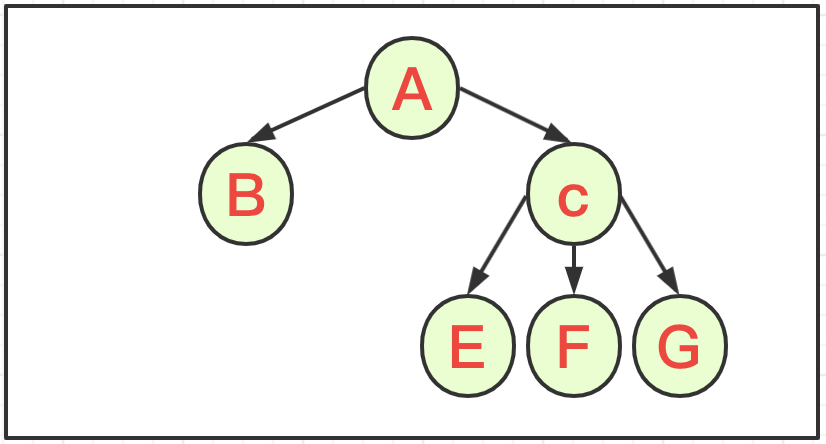# 五、源代码地址

GitHub·地址
GitEE·地址
https://gitee.com/cicadasmile/model-arithmetic-parent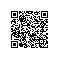使用钉钉扫一扫加入圈子
+ 订阅